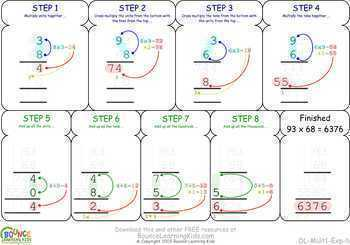# Multiplication (17 distance learning worksheets for Numeracy)Subject
Resource Type
File Type
Zip (10 MB|17 pages)
\$6.95
Also included in:
1. Addition, Subtraction, Multiplication & Division worksheets.Exhaustive range of basic operations covered within each individual set. Get the entire bundle for a terrific discount.AdditionSubtractionMultiplicationDivisionIdeal for distance learning - no prep required!Free TPT credits:Go to the My
\$20.80
\$13.95
Save \$6.85
Product Description

Series of multiplication worksheets. The entire range of multiplication arithmetic is handled. The worksheets start out simple and become progressively more challenging. Help sheets provide tutorials on how to multiple using step-by-step visual aids. Each worksheet has 16 multiplication sums to solve.

1. Multiplying by 0 or 1.
2. Multiplying numbers from 2 to 5.
3. Multiplying 10 by numbers from 0 to 9.
4. Multiplying 100 by numbers from 0 to 9.
5. Multiplying 10 by numbers 10 to 90 in increments of 10.
6. Tutorial sheet for how to multiply two 2-digit numbers when there are no numbers that need to be carried over.
7. Tutorial sheet for how to multiply two 2-digit numbers when there are numbers that need to be carried over.
8. Multiplying 100 by numbers from 1-99.
9. Multiplying single-digit and double-digit numbers together.
10. Multiplying 2 two-digit numbers together (with visual aids).
11. Multiplying 2 two-digit numbers together (visual aids progressively dropping off)
12. Multiplying three-digit and two-digit numbers together (visual aids progressively dropping off).
13. Multiplying four-digit and two-digit numbers together (visual aids progressively dropping off).
14. Multiplying two-digit and three-digit numbers together (visual aids progressively dropping off).
15. Multiplying three-digit and three-digit numbers together (visual aids progressively dropping off).
16. Multiplying four-digit and three-digit numbers together (visual aids progressively dropping off).

In this range:

Suitable for printing in B/W as well as colour.

Ideal for distance learning - no prep required!

Each worksheet is presented as an individual JPG but there is also a single PDF that contains all the worksheets together, so you have the best of both worlds to choose from.

Free TPT credits:

Go to the My Purchases page and find the Provide Feedback button. Use that to leave a rating/comment. Each time you do this, TPT will give you credits towards future purchases. As an added bonus, the feedback will help me to improve my products :-)

Half price:

Scroll to the top of this page and click on the green star just below the Bounce Learning Kids store name. You'll be able to get new products for half off in the first 24 hours of release!

FREE products:

More quality Visual Learning Resources from Bounce Learning Kids:

Total Pages
17 pages